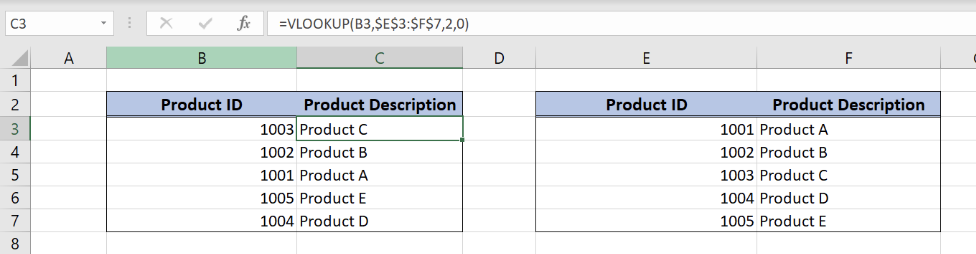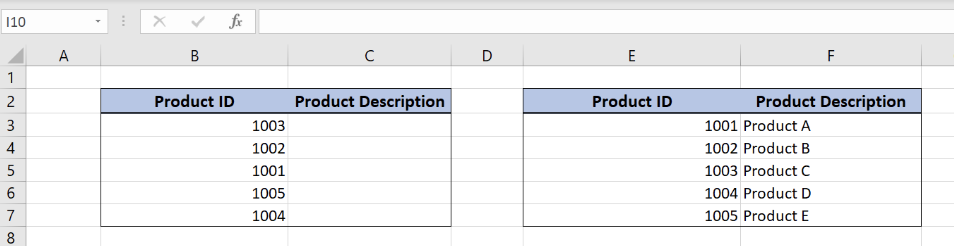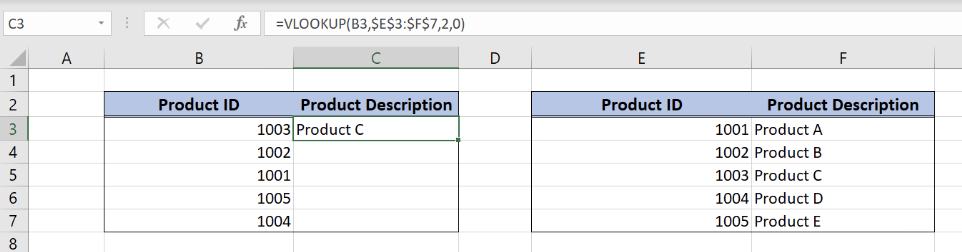Get instant live expert help with Excel or Google Sheets“My Excelchat expert helped me in less than 20 minutes, saving me what would have been 5 hours of work!”

#### Post your problem and you’ll get Expert help in seconds.

Your message must be at least 40 characters
Our professional Expert are available now. Your privacy is guaranteed.

# How to Copy a VLOOKUP Formula Down a Column

While using the VLOOKUP function in Excel, we sometimes need to apply it to a range. This is possible by using an absolute cell reference. In this tutorial, we will learn how to quickly copy a VLOOKUP formula down a column.Figure 1. Final result

## Syntax of the VLOOKUP formula

The generic formula for the VLOOKUP function is:

`=VLOOKUP(lookup_value, table_array, col_index_num, range_lookup)`

The parameters of the VLOOKUP function are:

• lookup_value – a value that we want to find in a table_array
• table_array – a range in which we want to lookup
• col_index_num – a column number in table_array from which we would like to pull a value
• range_lookup – default value 0. This means that we want to find an exact match for a lookup value.

## Setting up Our DataFigure 2. Data that we will use in the example

Let’s start with examining the structure of the data that we will use.

In the range B2:C7 we have the result table, while in the range E2:F7 we have the lookup table. Both tables consist of “Product ID” (columns B and E) and “Product Description” (columns C and F).

Get the Product description using VLOOKUP

Our goal is to obtain data from the “Product Description” column in the second table and populate it into the same column of the 1st table. This will be done based on each corresponding Product.

### Formula:

`=VLOOKUP(B3,\$E\$3:\$F\$7,2,0)`

In our example, the lookup_value is the individual cell in “Product ID” column. The parameter table_array is \$E\$3:\$F\$7 because we want to find value from the range B3:B7. Col_index_num has value 2, as we want to pull value from the second column of the range. Finally, range_lookup has value 0, because we want to find an exact match of “Product ID” values.Figure 3. Using the VLOOKUP function in the single cell

As a result, we will get Product C in the cell B3. As we can see, the value of “Product ID” in B3 in the 1st table is 1003, while in the 2nd table Product description for 1003 is Product C. The function pulls this value and returns it to the 1st table (cell C3) as a result.

Copying a VLOOKUP Formula using Absolute Cell References

The most important thing when copying the formula down a column is to understand the difference between relative and absolute cell reference. Excel uses relative cell reference when copying the formula to another cell. In our case, if we copy the formula from the cell B3 to the C3, the parameter lookup_value (B3) will be changed to C3. This is ok because we want to find a Product description for all the cells from the range. On the other hand, we want our VLOOKUP table (E3:F7) to be always the same while copying the formula. Here we must use the absolute cell reference and put \$ sign before rows and columns in the range (\$E\$3:\$F\$7).

To copy the formula down a column, we need to follow these steps:

• Select cell C3 and click on it
• Insert the formula: `=VLOOKUP(B3,\$E\$3:\$F\$7,2,0)`
• Press enter
• Drag the formula down to the other cells in the column by clicking and dragging the little “+” icon at the bottom-right of the cell.Figure 4. Copying the VLOOKUP function to the entire column

Most of the time, the problem you will need to solve will be more complex than a simple application of a formula or function. If you want to save hours of research and frustration, try our live Excelchat service! Our Excel Experts are available 24/7 to answer any Excel question you may have. We guarantee a connection within 30 seconds and a customized solution within 20 minutes.

Are you still looking for help with the VLOOKUP function? View our comprehensive round-up of VLOOKUP function tutorials here.

### Did this post not answer your question? Get a solution from connecting with the expert.Another blog reader asked this question today on Excelchat:
Solution examplesI have a pivot table in which per order (on the rows) stands how much products they ordered per size (on the columns). I want to determine which combinations of quantities of sizes people order. And I want to count these combinations.
Solved by E. W. in 60 minsI have 500 numbers in column A with aproximatly 15digits. In column B I have 5 numbers with 6 digits Can a find a formula wich can transfer all numbers from A column wich start with some 6 digitsa from B column
Solved by V. L. in 32 minsI have a sheet with 3 columns. First column is a code for records in column B (has 563 records). What I need is a formula to show me if what is in column C (has 4400 records) is in column B and if it is to take the code for that record. Example: Column A(code corresponding to column B): 12, 14, 15, 19 Column B(names): asd, adf, ade, aqw Column C(names): akd, adf, ade, anb, wgs I need something that would say, if record in column C (for example adf) is matching record in column B (adf) return the code from column A (the code corresponding to that record in column B),if not return blank.
Solved by C. J. in 8 minsIf the data in A matches the data in C, then I need the data in E to be placed in B
Solved by X. W. in 40 minsI have a workbook with 2 sheets. First sheet has column A which is a device name, then columns B - infinity that have numeric "tags" for that device. Each device can have one or 20 "tags", tags are all numerical. Worksheet 2 has column A which is numeric value and B which is a human readable value of what the tag is. Question is, how do I replace all the tag numbers in worksheet 1 with the actual human readable values is sheet 2?
Solved by T. H. in 16 mins## Subscribe to Excelchat.coAnother blog reader asked this question today on Excelchat: